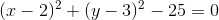# High School Math : How to find the equation of a circle

## Example Questions

### Example Question #1 : How To Find The Equation Of A Circle

The center of a circle is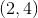and its radius is. Which of the following could be the equation of the circle?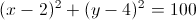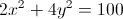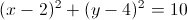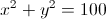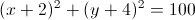Explanation:

The general equation of a circle is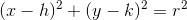, where the center of the circle is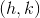and the radius is.

Thus, we plug the values given into the above equation to get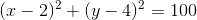### Example Question #1 : How To Find The Equation Of A Circle

Which one of these equations accurately describes a circle with a center of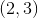and a radius of?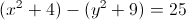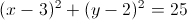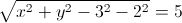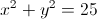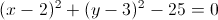Explanation:

The standard formula for a circle is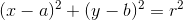, with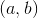the center of the circle andthe radius.

Plug in our given information.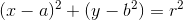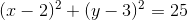This describes what we are looking for.  This equation is not one of the answer choices, however, so subtractfrom both sides.